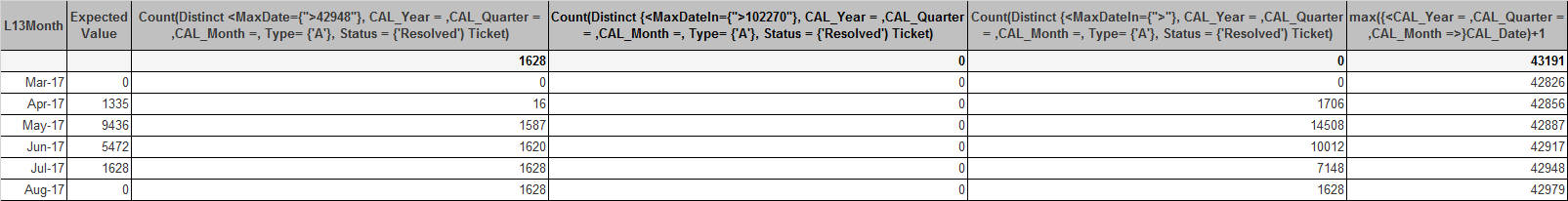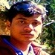# QlikView App Dev

Discussion Board for collaboration related to QlikView App Development.

Announcements
Welcome to Qlik Community! Check out our new navigation! FIND OUT MORE
cancel
Showing results for
Did you mean:Contributor II

## Formula/variable inside set analysis is not calculating as per the dimension in straight table

Hi Guys,

I'm making a straight table with CAL_Month as dimension and an expression to calculate the no of tickets resolved with a type 'A' and the calendar date is greater than the Maxdate using the below expression,

Count(Distinct <MaxDate={">\$(=max(CAL_Date)+1)"}, CAL_Year = ,CAL_Quarter = ,CAL_Month =, Type= {'A'}, Status = {'Resolved') Ticket)

This works fine in a text box where I display the value for one month at a time (I have Year & Month filters as well in the dashboard).

But when I try to display the same value in a line chart for all months (ignoring the Month & Year selections in the dashboard) its not working fine. Because the max date is not properly calculating at CAL_Month dimension level.

I have tried below three possibilities,

Exp 2:-

Count(Distinct <MaxDate={">\$(=max(CAL_Date)+1)"}, CAL_Year = ,CAL_Quarter = ,CAL_Month =, Type= {'A'}, Status = {'Resolved') Ticket)

Exp 3:-

Count(Distinct <MaxDate={">\$(=max({<CAL_Year = ,CAL_Quarter = ,CAL_Month =>}CAL_Date)+1)"}, CAL_Year = ,CAL_Quarter = ,CAL_Month =, Type= {'A'}, Status = {'Resolved') Ticket)

Exp 4:-

Count(Distinct <MaxDate={">\$(=aggr(max({<CAL_Year = ,CAL_Quarter = ,CAL_Month =>}CAL_Date)+1),CAL_Month)"}, CAL_Year = ,CAL_Quarter = ,CAL_Month =, Type= {'A'}, Status = {'Resolved') Ticket)

Exp 5:- Here I just pasted the max date calculation separately which is working fine, but the same is not working inside a set analysis calculation.

And these results as below,Thanks.

1 Solution

Accepted SolutionsPartner - Creator

Try using nested if conditions and forcefully join the dimension with your expression like below, this may be a stupid idea but this resolves this issue.

if(L13Month = 'Jan-2018',

Count(Distinct <MaxDate={">31-01-2018"}, CAL_Year = ,CAL_Quarter = ,CAL_Month =, Type= {'A'}, Status = {'Resolved') Ticket),

if(L13Month = 'Feb-2018',

Count(Distinct <MaxDate={">28-02-2018"}, CAL_Year = ,CAL_Quarter = ,CAL_Month =, Type= {'A'}, Status = {'Resolved') Ticket),

...etc.,

Considering you have only 13 values always (L13Month) you can make it work, but if the values start increasing then it may cost in performance of the dashboard. In which case we need to think of a dynamic way of doing it.

Thanks,

Shan S

10 RepliesMVP

Looks like you have MonthYear as dimension and you are trying to get for each month year max date.

Set Expression is working once per chart. Not once per line.

So here your Max(CAL_Date) is same for all MonthYear. Don't expect that you will get different result for MaxDate = {">\$(=max(CAL_Date)+1)"}

You can achieve this using Aggr and If function or creating flag for max date inside script.

Provide your sample data or sample app. We can try to help you in this case.Contributor II
Author

Hi Manish,

Thanks for your reply, yes you got my problem exactly.

I too tried using Aggr & if conditions like below, but I didn't get the result as expected.

if(date(aggr(max(CAL_Date)+1,CAL_MonthYear),'MM/DD/YYYY') < date(MaxDateIn,'MM/DD/YYYY'),
Count(DISTINCT {<CAL_Year = ,CAL_Quarter = ,CAL_Month =, Type= {'A'}, Status = {'Resolved'}>} Ticket),0)

Attached is the sample data I'm using for this, Please help.

Thanks.MVP

Provide sample data.. Would try to help you.Contributor II
Author

I'm trying but not able to upload the sample data, not sure what's the issue. Is there a way to upload it.MVPPartner - Champion III

I expect that the problem is the DISTINCT. In a text box, it will count distinct value across the entire model, and in the chart, it will take distinct values for the current month, but a value could be in multiple months. The solution is an Aggr() expression:

Sum(Aggr(

Count(Distinct <MaxDate={">\$(=max(CAL_Date)+1)"}, CAL_Year = ,CAL_Quarter = ,CAL_Month =, Type= {'A'}, Status = {'Resolved') Ticket), L13Month)

(assumes that you have a single dimension in the chart, L13Month)

Logic will get you from a to b. Imagination will take you everywhere. - A EinsteinContributor II
Author

Hi Manish,

Thanks for your help, I think there is some firewall block in my network, I'm uploading a sample here from my PC.In this sample data I have covered all my scenarios.

As per this sample data below is my expected results both in text object and Line chart. And the issue is its working fine in Text object but not in Line chart since the Max(CAL_date) is not getting calculated at Line level (Month Year level).

 CAL_Year CAL_Month CAL_Quarter L13M Max(CAL_Date) Expected result both Text object & Line chart Comments May 2017 Q1 17-May 5/31/2017 8 MaxDate > Max(CAL_Date)+1 which means tickets resolved from June onwards with Type = 'A' June 2017 Q1 17-Jun 6/30/2017 6 MaxDate > Max(CAL_Date)+1 which means tickets resolved from July onwards with Type = 'A' July 2017 Q2 17-Jul 7/31/2017 3 MaxDate > Max(CAL_Date)+1 which means tickets resolved from August onwards with Type = 'A'Contributor II
Author

Hi Jonathan,

I have already tried using sum(aggr()) at month year level (That's the only dimension I have in Chart), but still I can see the Max(CAL_Date) is calculating at overall datamodel level and not at Line level/aggr(L13Month) level.

Is there a way to force it to calculated at Line level.

Thanks.Partner - Creator

Try using nested if conditions and forcefully join the dimension with your expression like below, this may be a stupid idea but this resolves this issue.

if(L13Month = 'Jan-2018',

Count(Distinct <MaxDate={">31-01-2018"}, CAL_Year = ,CAL_Quarter = ,CAL_Month =, Type= {'A'}, Status = {'Resolved') Ticket),

if(L13Month = 'Feb-2018',

Count(Distinct <MaxDate={">28-02-2018"}, CAL_Year = ,CAL_Quarter = ,CAL_Month =, Type= {'A'}, Status = {'Resolved') Ticket),

...etc.,

Considering you have only 13 values always (L13Month) you can make it work, but if the values start increasing then it may cost in performance of the dashboard. In which case we need to think of a dynamic way of doing it.

Thanks,

Shan S